# K-Nearest-Neighbors Classification Algorithm in Practice

What the KNN algorithm is and how it is used in classification tasks.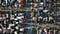Photo by Tom Rumble on Unsplash

# Introduction

KNN algorithm is a supervised classification algorithm that is mainly used to predict which category a query point belongs to, given a bunch of data values with respect to its corresponding categories (class labels). Talking from the perspective of the classification tasks, KNN is very much similar to Naive Bayes but slightly different in terms of technicality and implementation. In Naive Bayes, we compute the likelihood by probabilistic methods, whereas in KNN, we compute distance measurements along with other extensions added.

One great advantage of KNN is…

# Naive Bayes Classification Algorithm in Practice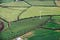Photo by Annie Spratt on Unsplash

# Introduction

Classification is a task of grouping things together on the basis of the similarity they share with each other. It helps organize things and thus makes the study more easy and systematic. In statistics, classification refers to the problem of identifying to which set of categories an observation or data value belongs to.

Examples -

• Identifying whether an email is a spam or not spam.
• On certain parameters of health, identifying or classifying whether a patient gets a heart attack or not.

For humans, it can be very easy to do the classification task assuming that he/she has proper domain-specific…

# Crimes Against Women — Geo Data Analysis

In this article, we will see the complete analysis of “Crimes Against Women” that took place in India from 2001 to 2014.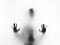Photo by Stefano Pollio on Unsplash

# Introduction

The main agenda of this article to analyze crime data by following all the steps required for data analysis. The steps include Data Preparation, Data Cleaning, Data Wrangling, Feature Selection, Data Visualization & Comparison.

The data is about the crimes committed against women in India. The data is being recorded from 2001 to 2014. It includes crimes like -

• Rape
• Kidnapping and Abduction
• Dowry Deaths
• Assault on Women with intent to outrage her modesty
• Insult to the modesty…

# Convert a Regular Matrix into Sparse Matrix in Python

In this article, we will step by step procedure to convert a regular matrix into a sparse matrix easily using Python.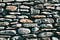Photo by Marco Pregnolato on Unsplash

# Introduction

Matrix is a type of data structure similar to an array where values are stored in rows and columns. Here, the values are of a unique type. When dealing with matrices (linear algebra) in Machine Learning and NLP, we often hear about two types of matrices as -

• Dense Matrix — The matrix where most of the elements are non-zero. In this matrix, there are very few zero elements.
• Sparse Matrix — In contrast, the matrix where most of…

# Matrix Multiplication — Normal Function to an Optimised Code

In this article, we will learn different ways of multiplying matrices from an easy-to-read function to an optimized code.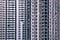Photo by Carl Nenzen Loven on Unsplash

# Introduction

If you had read my previous articles on matrix operations, by now you would have already know what a matrix is. Yes, a matrix is a `2D` representation of an array with `M` rows and `N` columns. The shape of the matrix is generally referred to as dimension. Thus the shape of any typical matrix is represented or assumed to have (`M` x `N`) dimensions.

• Row Matrix — Collection of identical elements or objects stored in `1` row and `N` columns.

# How to Deploy a Python Image Processing App

In this article, I will share my experience (the errors and issues) while deploying the app which I developed.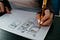Photo by Kelly Sikkema on Unsplash

For a while till now, I have been working on my basic image processing app developed in Python using the frameworks and libraries like -

• Dash
• Plotly
• Plotly express
• NumPy &
• OpenCV
• and some other dependencies

Let me explain how the journey of developing this app began.

# Idea

First, I didn’t have any idea or plan to develop an app (that too for image processing). It is when one of my colleagues asked in our common group -

How do I re-mirror…

# Transposing a Matrix — Normal Function to the Optimised Code

In this article, we will learn 3 ways of transposing a matrix from an easy to read function to an optimized code without needing any for loops.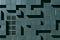Photo by Vlado Paunovic on Unsplash

# Introduction

A matrix is a 2D representation of an array with M rows and N columns. An array is a collection of identical elements or objects stored in 1 row and N columns. There are so many mathematical operations and properties that can be implemented in a matrix. One such operation is the transpose operation. Transposing a matrix is easy, just converting rows into columns and vice-versa.

# How to find an Intersection between two matrices easily using NumPy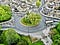Photo by Benjamin Elliott on Unsplash

# Introduction

The word intersection in mathematics is termed as the similar (smaller) objects between two different objects. Intuitively, we can say the intersection of objects is that it belongs to all of them.

Geometrically speaking, if we have two distinct lines (assuming these lines are two objects), the intersection of these two lines would be the point where both the lines meet. Well, in the case of parallel lines, the intersection doesn’t exist. Geographically, the common junction between two or more roads can be taken as the area or region of intersection.

In Set theory, the intersection of two objects such…

# Complete Understanding of Morphological Transformations in Image Processing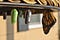Photo by Suzanne D. Williams on Unsplash

In the previous articles on morphological transformations, we learned the two important transformations namely erosion and dilation. In this article, we will implement the other transformations which are built on top of these two. They are -

• Opening
• Closing
• Morphological Gradient
• Top hat
• Black hat
• Boundary Extraction
• Hit — Miss Transformation

We have seen a step-by-step implementation of erosion and dilation explaining the convolution method with simple matrix operations. In all of these transformations, we rely on the binary input image, structuring element, or kernel. The structuring element needs to be a square matrix which is again a binary matrix.

# The Math Behind Image Dilation, Explained With Python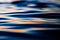Photo by Jason Leung on Unsplash

Like Image Erosion, Image Dilation is another important morphological operation used to increase or expand shapes contained in the input image. Think of this as “ diluting ” the image. Diluting anything requires water, here we need a structuring element or kernel.

Note: We are not expanding or increasing the image size. We are increasing the pixel strength and the size remains the same.

Mathematically, we can represent this operation in the following way -## Sameer

Python Developer | Python Mentor | Aspiring Geo Data Scientist | Support me: https://www.buymeacoffee.com/msameeruddin